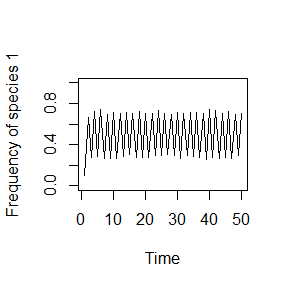## Online Box 4: R code for simulating delayed negative frequency-dependent selection: Figure 6.5 in the book

“Delayed” means that fitness is fixed for an entire “year”, thus requiring two time loops instead of one. Only one simulation is run here (i.e., there is no loop over num.sims)

## specify parameters, initial conditions, and output vector
num.years <- 50
freq.1.vec <- vector(length = num.years)

J <- 500
init.1 <- 0.1*J
COM <- vector(length = J)
COM[1:init.1] <- 1; COM[(init.1+1):J] <- 2

year <- 2

fit.ratio.avg <- 1
freq.dep <- -20

## record data (frequency of species 1) for year 1
freq.1.vec <- sum(COM==1)/J

## run simulation
for (i in 1:(num.years-1)) {

freq.1 <- sum(COM==1)/J; freq.2 <- 1 - freq.1
fit.ratio <- exp(freq.dep*(freq.1-0.5) + log(fit.ratio.avg))
Pr.1 <- fit.ratio*freq.1/(fit.ratio*freq.1 + freq.2)

for (k in 1:J) {
COM[ceiling(J*runif(1))] <- sample(c(1,2), 1, prob=c(Pr.1,1-Pr.1))
}

## record data
freq.1.vec[year] <- sum(COM==1)/J
year <- year + 1
}

## graph the results
plot(1:num.years, freq.1.vec, type="l", xlab="Time",
ylab="Frequency of species 1", ylim=c(0,1))### Description of the code.

1. This is the start of the first time loop, one loop for each year. freq.1 at the start of the year is used to calculate fit.ratio and Pr.1, which will remain constant for the whole year (a period of J deaths).
for (i in 1:(num.years-1)) {

freq.1 <- sum(COM==1)/J; freq.2 <- 1 - freq.1
fit.ratio <- exp(freq.dep*(freq.1-0.5) + log(fit.ratio.avg))
Pr.1 <- fit.ratio*freq.1/(fit.ratio*freq.1 + freq.2)
1. This is the second time loop, one loop for each of J deaths.
  for (k in 1:J) {
COM[ceiling(J*runif(1))] <- sample(c(1,2), 1, prob=c(Pr.1,1-Pr.1))
}